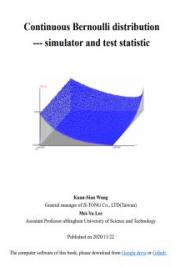## Continuous Bernoulli distribution---simulator and test statistic - PDF Preview# Continuous Bernoulli distribution---simulator and test statistic

This is an advanced and completely descriptive book for the continuous Bernoulli distribution that is very important to deep learning and variational autoencoder. This book is free to read and contains (1) the continuous Bernoulli distribution about sufficient statistic, point estimator, test statistic, confidence interval, the goodness of fit, and one-way analysis. (2) The test statistic and confidence interval of two Continuous Bernoulli populations. (3) The Continuous Trinomial...